### Calculus Fundamentals

In the last quiz, we looked at some examples of limits. Here is the general idea.

We say that the limit of the function $f$ as $x$ approaches $a$ is the number $L$ if, as $x$ gets closer and closer to $a,$ the function values $f(x)$ get closer and closer to $L.$ If there is no such number $L,$ we say the limit does not exist.

When the limit exists, we use the notation $\lim\limits_{x \to a} f(x) = L.$

In this picture, for example, the limit of the function (in blue) as $x$ approaches 2 (from either side) is 4.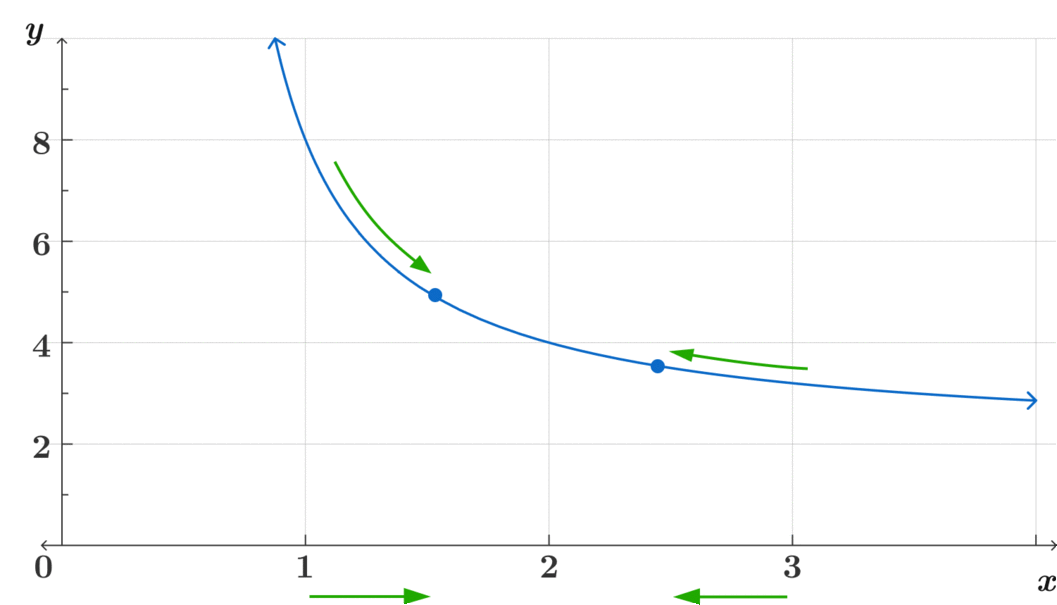As the input approaches 2, the output approaches 4.

In some ways this is a simple idea, but as we’ll see, there are plenty of subtleties involved!

# Limits of Functions

Let’s start with a straightforward example. Here’s a graph of a function $f.$ What is the limit of $f(x)$ as $x$ approaches 1? In other words, as the input gets closer and closer to 1, what value is the output getting closer to?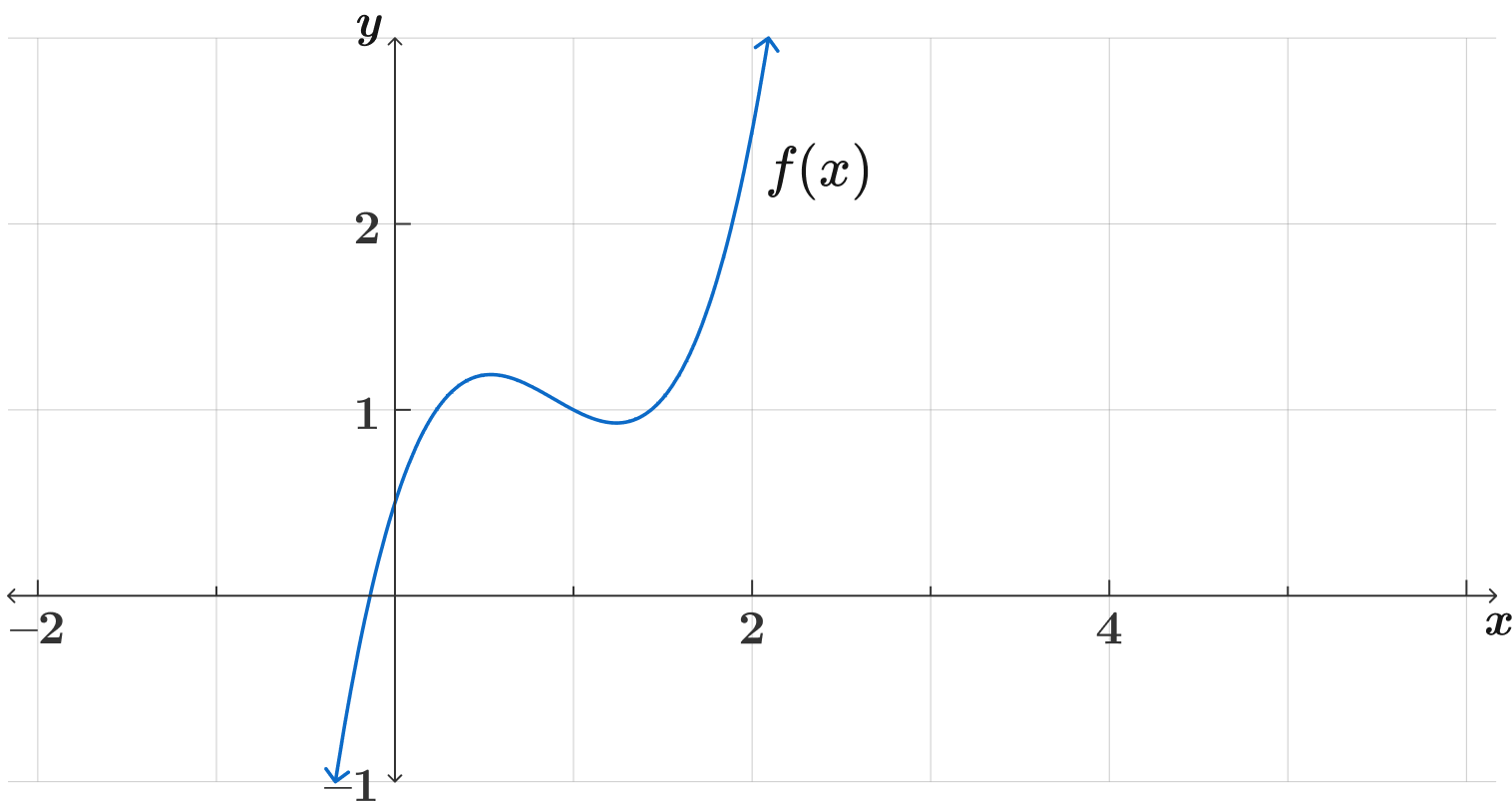# Limits of Functions

Now consider the function $f$ given by the formula:

$f(x) = \begin{cases}\begin{array}{rl} x^2, & x \neq 1 \\ 3, & x = 1 \\ \end{array}\end{cases}$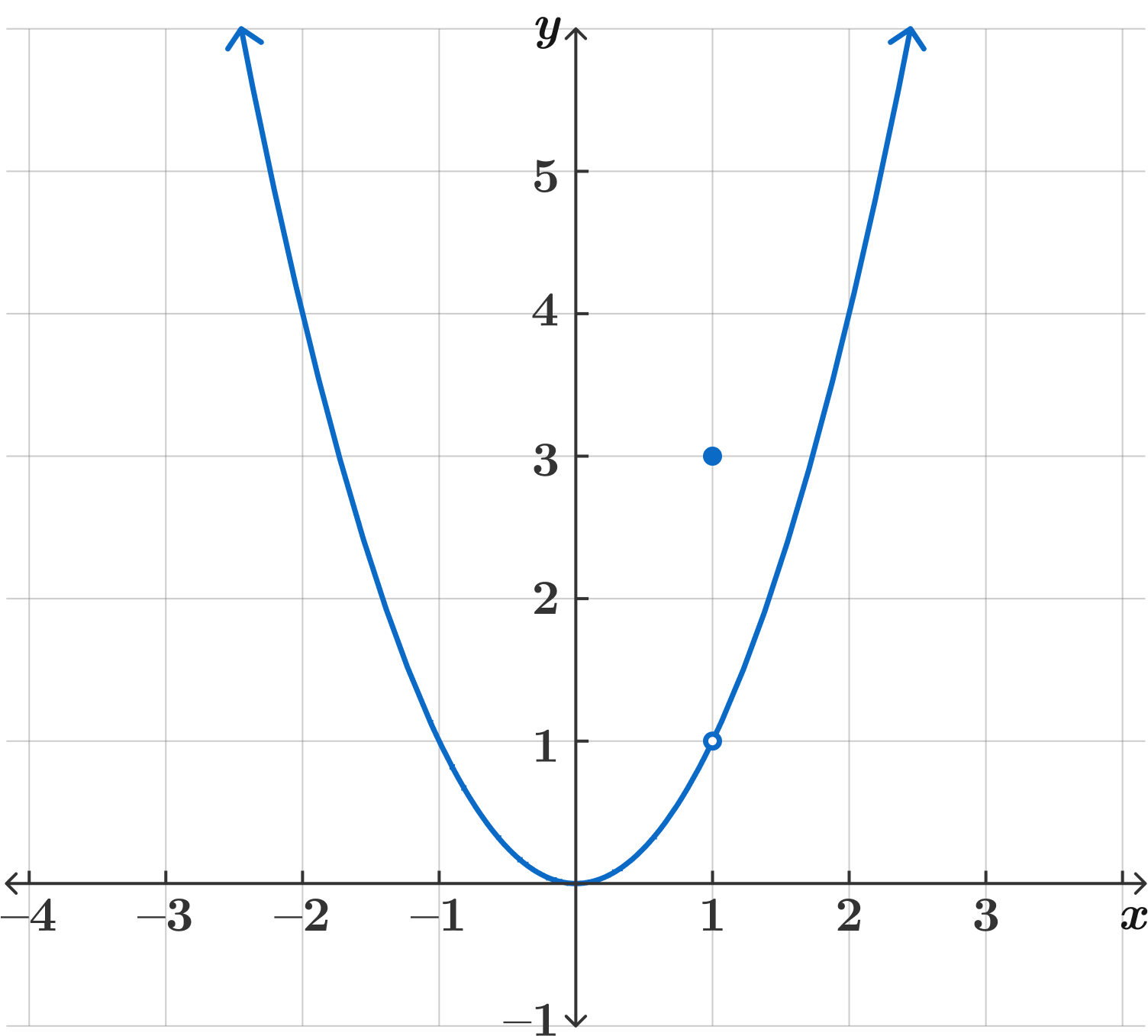In other words, $f$ is the usual function $y=x^2,$ except that we’ve set the value at $x=1$ to be 3. What is $\lim\limits_{x \to 1} f(x)$?

# Limits of Functions

In the previous example, the value of the function at 1 was 3. But the limit was still 1, because as the $x$ values get closer and closer to 1, the function values get closer and closer to 1. This is an important point:

$\lim\limits_{x \to a} f(x)$ has nothing to do with the value of $f$ at $a$ itself! It only says something about what happens as $x$ gets close to $a$.

# Limits of Functions

Here’s another interesting example. Define

$f(x) = \begin{cases}\begin{array}{rl} -1, & x \leq 0 \\ 1, & x > 0 \\ \end{array}\end{cases}$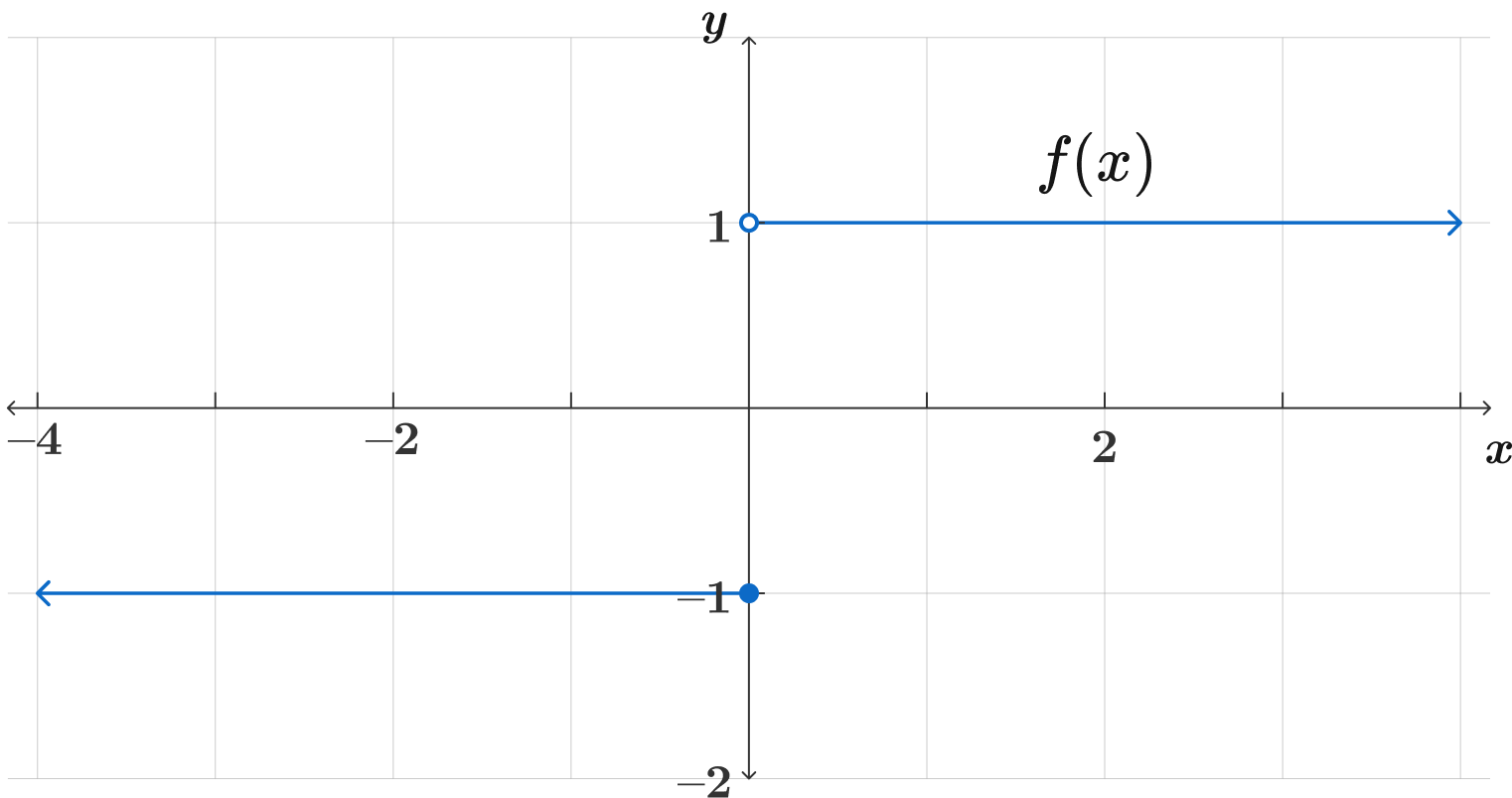What is $\lim\limits_{x \to 0} f(x)$?

Note: For this to exist, the value of the function, $f(x),$ must be getting closer to some number $L$ as $x$ gets closer to 0… no matter how close $x$ gets to 0.

# Limits of Functions

This is our first example in this quiz of a limit that doesn’t exist. It’s true that as $x$ approaches 0 from the right, the function values approach 1. And as $x$ approaches 0 from the left, the function values approach -1. But this means there’s no single $L$ that the function approaches no matter how close $x$ gets to 0. So the limit doesn’t exist.

This example, where the "right-hand" (as $x$ approaches from the right) and "left-hand" (as $x$ approaches from the left) limits exist but aren’t equal, is the simplest way a limit might not exist. But there are many other ways. For example, in the previous quiz we saw that $\lim\limits_{x \to 0} \sin\left(\frac{1}{x}\right)$ does not exist, because as $x$ gets small, $\frac{1}{x}$ gets large, and so $\sin$ just oscillates between -1 and 1, instead of approaching any particular $L.$

# Limits of Functions

The function $f(x),$ shown below, is defined on the interval $(0,9].$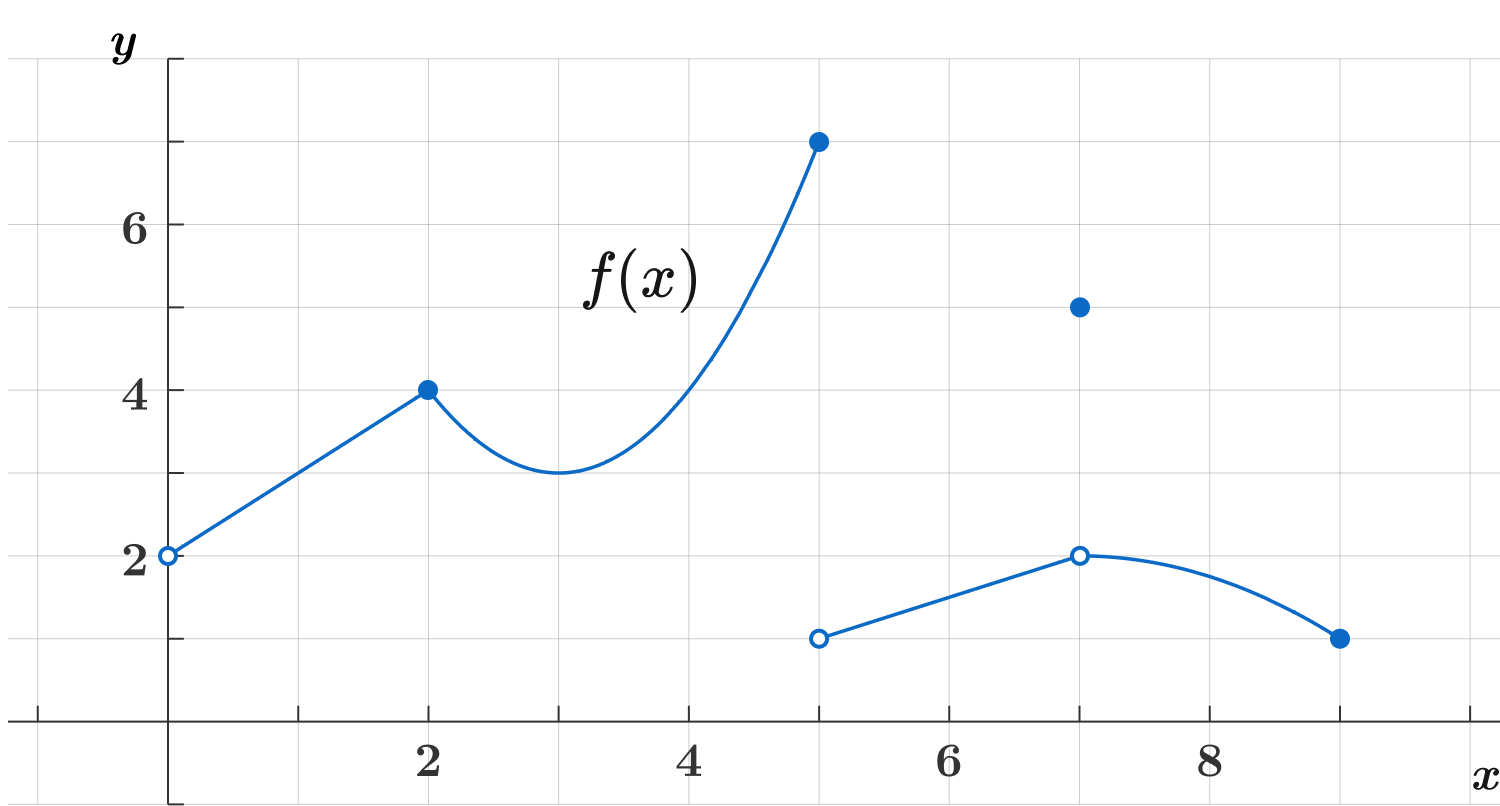How many of the limits below exist?

• $\displaystyle \lim_{x \rightarrow 2} f(x)$

• $\displaystyle \lim_{x \rightarrow 3} f(x)$

• $\displaystyle \lim_{x \rightarrow 5} f(x)$

• $\displaystyle \lim_{x \rightarrow 7} f(x)$

# Limits of Functions

Usually when we need to compute a limit in calculus, we won’t be presented with a graph, but with an algebraic expression. For example, let

$f(x) = \frac{x^2+2x-8}{x-2}$

What is $\lim\limits_{x \to 3} f(x)?$

# Limits of Functions

The last example was easy, because everything was well-behaved at $x=3.$ (In a later quiz, we’ll see this happens whenever the function is continuous.) Now consider:

$\lim_{x \to 2} \frac{x^2+2x-8}{x-2}$

The function is undefined at $x=2,$ because of the denominator. We simply cannot evaluate $f(2).$ But we can still investigate the limit as $x$ approaches 2, because that only depends on what $f$ is doing near 2, not at 2. In fact, notice that the numerator is also 0 when you plug in 2. This is another example of a $\frac{0}{0}$ indeterminate form from the first chapter. When we encounter such a thing, the limit is not obvious. Often though, we can discover it by algebraic manipulation.

What is the limit? (Hint: factor the numerator.)

# Limits of Functions

For the last three questions, we’re going to look at a strange and interesting example. The same basic idea behind limits hasn’t changed: $\lim\limits_{x \to a} f(x) = L$ means that as $x$ approaches $a,$ the values $f(x)$ approach $L.$

Define:

$f(x) = \begin{cases}\begin{array}{rl} x, & \text{if } \; x = \frac{1}{n} \text{ where } n \text{ is an integer } \\ 0, & \text{otherwise.} \\ \end{array}\end{cases}$

For example, $f(\frac{1}{2}) = \frac{1}{2},$ $f(0) = 0,$ and $f(\frac{2}{3}) = 0.$

Try to get a feel for what this function looks like. There will be a picture on the next page, but see if you can work it out without looking.

# Limits of Functions

Let's look at a function $f$ that is 0 almost everywhere except for $\frac{1}{n}$ points, which lie on the line $y=x$.

Part of the graph of $f$ looks something like this: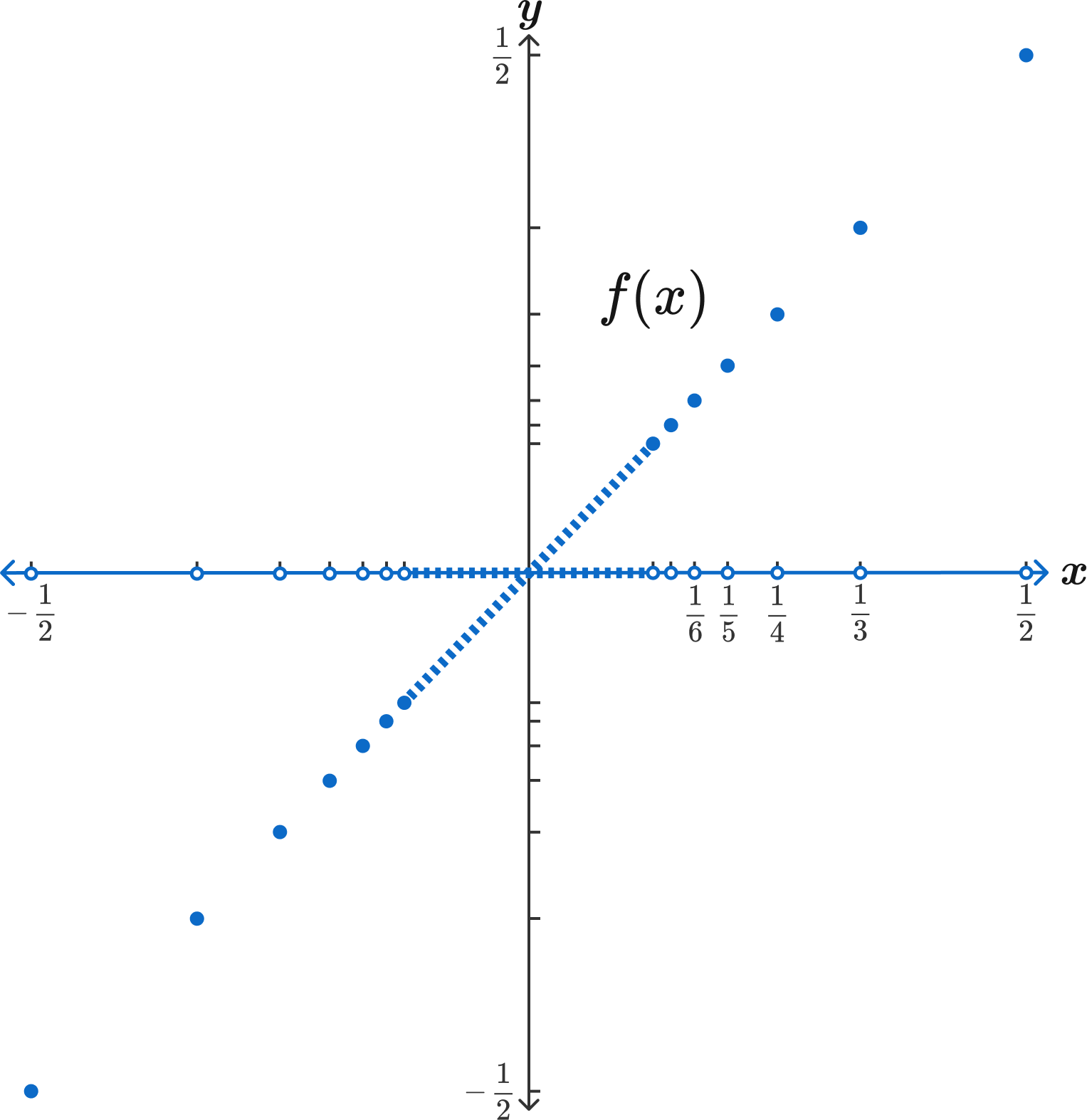Now, what is the limit of $f(x)$ as $x$ approaches $\frac{3}{7}$?

# Limits of FunctionsThe value of $f$ is 0, except for at $\frac{1}{n}$ points, which lie on the line $y=x$.

What about $\displaystyle\lim_{x \to \frac{1}{3}} f(x)\ ?$

# Limits of FunctionsThe value of $f$ is 0, except for at $\frac{1}{n}$ points, which lie on the line $y=x$.

Now for the most interesting question. What is $\lim\limits_{x \to 0} f(x)\ ?$

It’s hard to visualize exactly what’s happening near 0. But you know the rule: the function value is 0, except at points like $\frac{1}{2},$ $\frac{1}{3},$ $\frac{1}{4},$ etc. So as $x$ gets smaller and smaller, what happens to the $f(x)$ values?

# Limits of Functions

×